# M7-S5: Diffraction of Light### What is diffraction?

When light passes through an aperture, slit or around a bend/edge of an object, it experiences a scattering process called diffraction.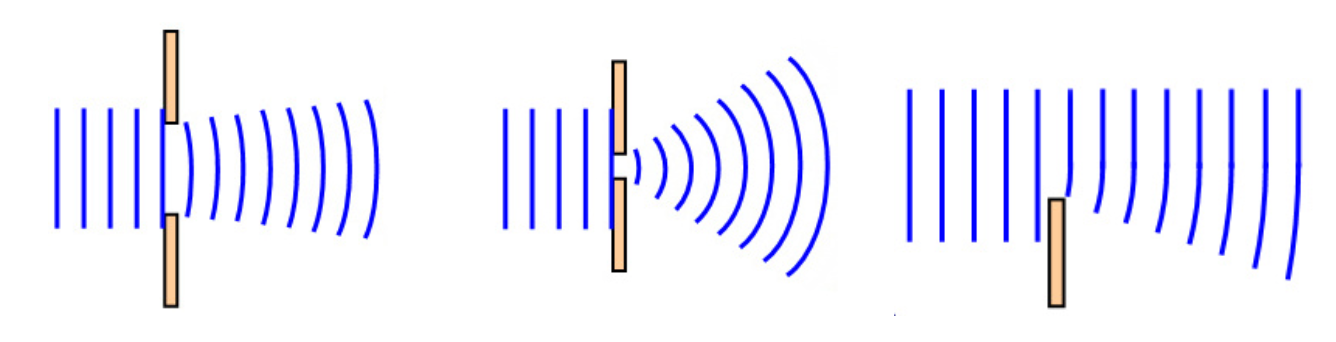• The scattering process bends light’s direction of propagation. The degree of bend is dependent on the relative difference between its wavelength and size of the slit.
• If the slit size is smaller than or equal to light’s wavelength, considerable diffraction occurs. Smaller the slit, greater the degree of diffraction.
• If the slit size is larger than light’s wavelength, diffraction still occurs but is unnoticeable to the naked eye.
• Examples of diffraction can be seen in all types of waves, including all electromagnetic waves, sound waves or waves observed in water.• Diffraction of light creates a unique repeating pattern

Diffraction of light creates a repeating pattern where a white band is observed centrally and bands of rainbow are dispersed away from the centre, each separated by dark space.

The ‘resolution’ of each rainbow band become more diffuse and weaker the further away it from the central white band.

### Huygens' Wave Model of Light

• Why does diffraction occur? – Huygens’s Principle
Huygens’s principle is commonly used to visualize the effect of diffraction. In the 17th century, Christiaan Huygens proposed that when a wave reaches an opening, its wavefront can be perceived as individual points emitting spherical secondary waves. Huygens’ theory explains the shape observed when a typical wave e.g. water wave propagates through an opening within a barrier. It also gives a plausible explanation to why waves can diffract around an edge of an opaque material.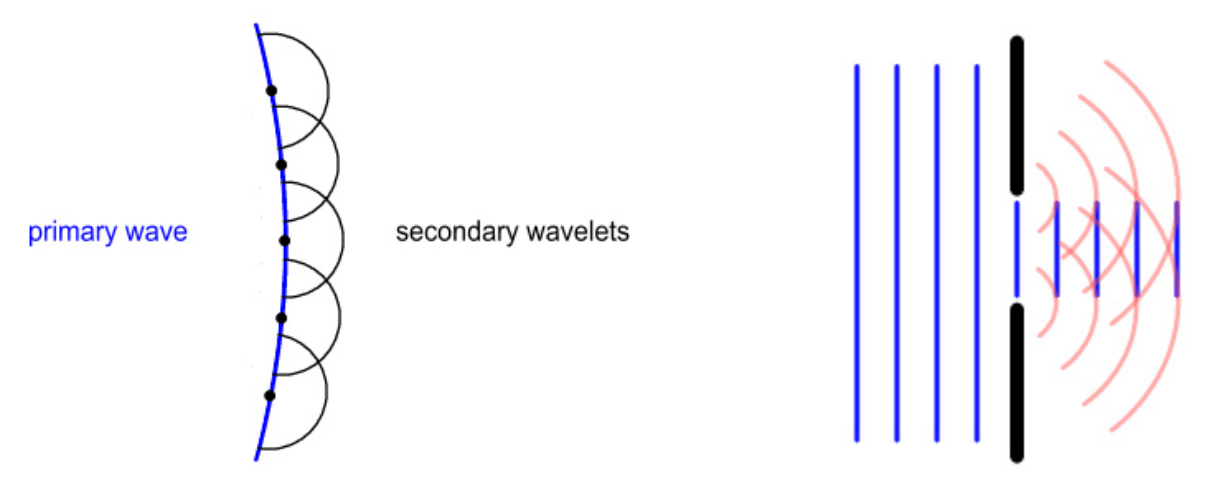However, his theory contained many flaws and did not address several important observations including:

• Why does the secondary spherical wave only propagate forward? In other words, why does it have a hemispherical shape instead of a full sphere.
• Why do secondary ‘wavelets’ cancel each other out to produce gaps between bands, observed in the diffraction of white light?
• What happens to the original primary wave after secondary ‘wavelets’ are emitted?

### Why does diffraction of light create the scattered bands?

In 1818, Fresnel improved Huygens’s theory by adding his theory of interference. Together, their theories could explain both the effect of diffraction and presence of dark fringes. Fresnel proposed that the diffraction pattern obtained from white light is a result of constructive and destructive interference between secondary ‘wavelets’ emitted from the original wavefront.

• When waves are out of phase, they undergo destructive interference. This results in dark fringes or ‘minima’ of diffraction.
• When waves are in phase, they undergo constructive interference. This results in spectral bands or ‘maxima’ of diffraction.The intensity of each spectral band decreases away from the centre. The intensity of each band indicates the relative amount of light hitting a particular region of the detector.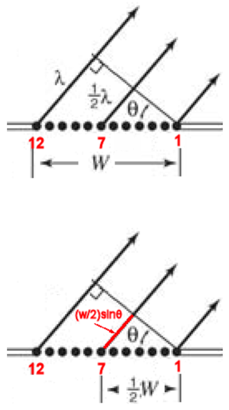• In the single-slit experiment, the Huygens-Fresnel principle can be quantitatively demonstrated. The diagram on the right illustrates an incident wave hitting a slit of width W, at a particular angle θ. Note that the wave can also propagate in other directions as Huygens’ wavelet is hemi-spherical in nature.
• These wavelets are propagating with equal velocity which means their relative position can lead to interference pattern.
• If the difference between wave 1 and 7 is exactly half a wavelength (l/2), they are out of phase and therefore will undergo destructive interference.
• Under the same circumstance, wavelets emitted from points 2 & 8, 3 & 9 etc. would also experience destructive interference.
• Thus, destructive interference (dark fringe) would occur at an angle θ for a given W and λ

(W/2)sinθ = λ/2 or Wsinθ = λ

• Since multiple dark fringes can be created at multiple locations due to presence of numerous wavelets, the product between slit width and angle can be perceived as a multiple of wavelength

Wsinθ = mλ        where m is an integer e.g. 1, 2, 3…

For the first dark fringe away from the centre, m = 1 so: Wsinθ = λ

• Note that, when W < λ, the equation has no solution. This means when the width of the slit/opening is smaller than the wavelength of light, no dark fringes would be observed at the ‘first’ location.The pattern generated from constructive and destructive interference can be also observed when light diffracts through multiple slits or apertures.

### Young's Double-slit Experiment

In 1801, Thomas Young conducted an experiment using a double-slit apparatus to support the wave model of light.

• If light was a particle or consisted of particles, only light particles which pass through the slits would be observed on the viewing screen• However, Young’s observation showed otherwise as it clearly demonstrated diffraction pattern like with a single-slit apparatus. In his experiment, monochromatic (single-colour) light was shone through two slits producing multiple bright bands/spots on the viewing screen rather than just two.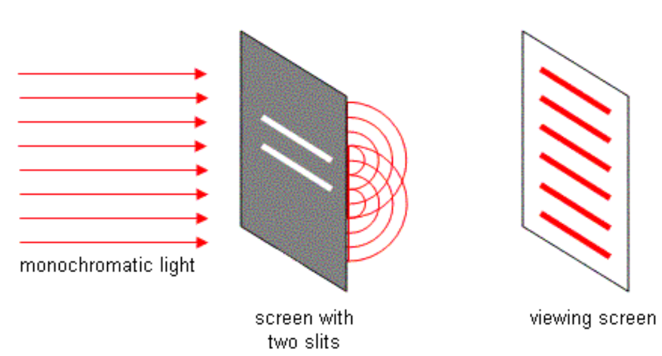• Young’s qualitative observations showed that light cannot be particles as they cannot diffract. In addition, the bright spots showed varying intensity and their position can be calculated for a given wavelength of light.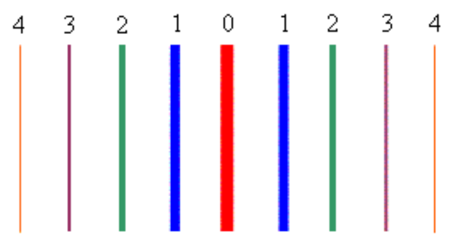Colours are used to demonstrate differences in intensity only. Monochromatic light would produce bands of the same colour.

• The position of each band can be quantitatively analysed.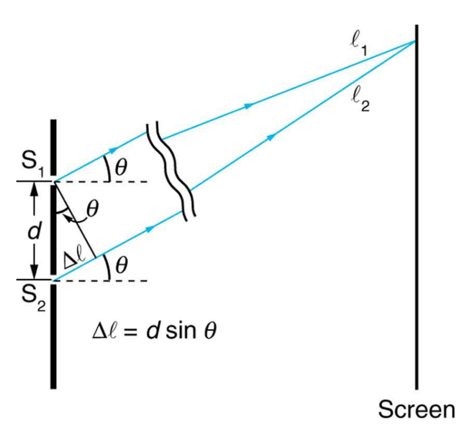• When the difference in phase between two waves are exactly one wavelength apart, they will experience constructive interference.Where d is the distance between two slits and m is the ‘order’ of interference.

• When the difference in phase between two waves are exactly half a wavelength apart, they will experience destructive interference.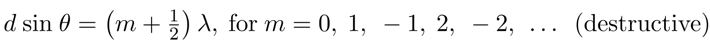Where d is the distance between two slits and m is the ‘order’ of interference.

• The order of interference begins with 0 which corresponds to the central band on the viewing board. The intensity of band decreases with higher orders of interference.

Suppose you pass light from a He-Ne laser through two slits separated by 0.0100 mm and find that the third bright line on a screen is formed at an angle of relative to the incident beam. What is the wavelength of the light?• Diffraction of light produces a similar array of bright and black spots when more than 2 slits are used.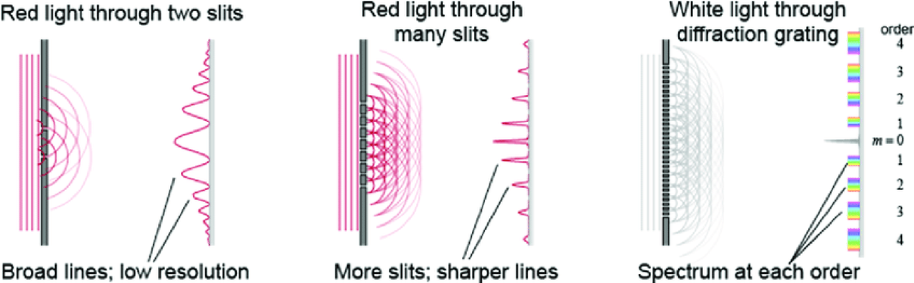• Increased number of slits produces diffraction bands with greater resolution.
• This is because as the number of slits increases, so does the number of waves being directed at the same angle (or same position on the viewing board).
• This increases the intensity of bright spots as more waves experience constructive interference which leads to a greater amplitude.
• In contrast, when waves do not exactly overlap at their crests or troughs, they will undergo destructive interference. The extent of destructive interference again increases with the number of slits. Greater the extent, more defined the black fringes are.

• White light produces spectra of visible light during diffraction. Within each spectrum, longer wavelength of visible light (red) is further away from the centre. This is because as l becomes larger, so does the angle.• For a particular wavelength of light and distance (d) between slits, the number of orders (m) of bright spots is finite. This can also be calculated: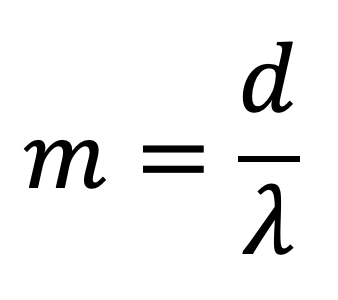• The greatest angle of bright spots is 90º away from the centre as this gives sine the greatest value of 1. By substituting the angle as 90º, we obtain the above equation which can be used to calculate the maximum order for a given distance between slits and wavelength of light.

#### How can we change the number of bright spots present?

• For a given wavelength, we can increase the separation distance between slits. However, this does not always work as when separation distance becomes too large, the interference between waves becomes negligible. This results in two bright spots being formed for a double slit experiment.
• For a given separation distance, we can decrease the wavelength of light to increase the number of maxima present.
• Both slit separation distance and wavelength can be manipulated to produce varying number of bright spots and dark fringes.

Practice Question 1 (3 marks)

Outline Huygens' model of light and explain how his model provided an understanding of diffraction.

Practice Question 2 (1 mark)

At what angle is the first-order maximum for 450 nm wavelength blue light falling on double slits separated by 0.05 mm?

Practice Question 3

A monochromatic light of wavelength 600 nm is passed through two slits 0.01 mm apart.

(a) Calculate the angle at which the second order maximum (bright spot) would be observed as a result of diffraction. (1 mark)

(b) How many orders of maxima are present when the light diffracts through the two slits? (1 mark)

(c) Hence, determine how many bright spots are expected to be present on the viewing board. (1 mark)

Previous section: Applications of Spectroscopy

Next section: Particle and Wave Models of Light